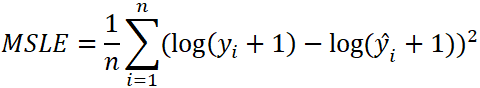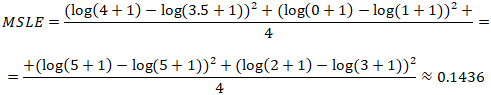# Calculate Mean Squared Logarithmic Error using TensorFlow 2

Mean squared logarithmic error (MSLE) is a loss function that is used to solve regression problems. MSLE is calculated as the average of the squared differences between the log-transformed actual and predicted values.

The formula to calculate the MSLE:• `n` - the number of data points.
• `y` - the actual value of the data point. Also known as true value.
• `ŷ` - the predicted value of the data point. This value is returned by model.

Let's say we have the following sets of numbers:

Here is example how MSLE can be calculated using these numbers:TensorFlow 2 allows to calculate the MSLE. It can be done by using `MeanSquaredLogarithmicError` class.

``````from tensorflow import keras

yActual = [4, 0, 5, 2]
yPredicted = [3.5, 1, 5, 3]

msleObject = keras.losses.MeanSquaredLogarithmicError()
msleTensor = msleObject(yActual, yPredicted)
msle = msleTensor.numpy()

print(msle)``````

MSLE also can be calculated by using `mean_squared_logarithmic_error`, `msle` or `MSLE` function.

``````msleTensor = keras.losses.mean_squared_logarithmic_error(yActual, yPredicted)
msle = msleTensor.numpy()

msleTensor = keras.losses.msle(yActual, yPredicted)
msle = msleTensor.numpy()

msleTensor = keras.losses.MSLE(yActual, yPredicted)
msle = msleTensor.numpy()``````